Home > CCG > Chapter 12 > Lesson 12.2.3 > Problem12-92

12-92.

Find the area and perimeter of the shape at right. Assume that any non-straight portions of the shape are part of a circle. Show all work. Homework Help ✎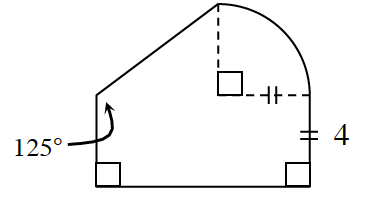Break up the shape into smaller, more manageable shapes.
There is more than one way to do this.

$125^\circ − 90^\circ = 35^\circ$

Fill in all known angles and lengths.

Use trigonometry to find the length of the triangle's unknown leg.

$\tan35^\circ = \frac{4}{x} \text { or } \frac{\sin 55^\circ}{x} = \frac {\sin 35^\circ}{4}$

Find the area of each the smaller shapes.

How does each of these smaller areas relate to the area of the larger shape?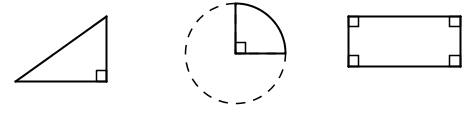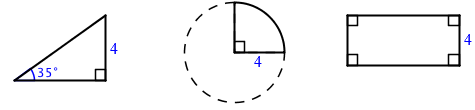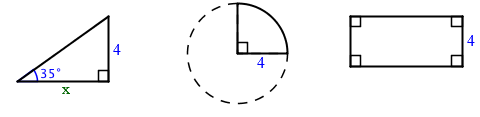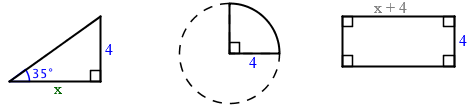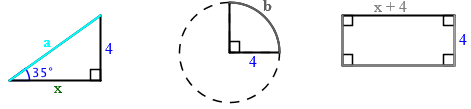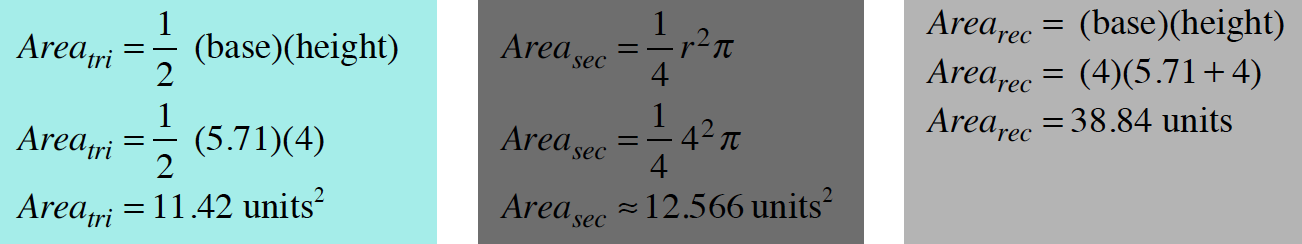Find the lengths of the sides that form the perimeter
of the original shape. Start with the hypotenuse of
the triangle.

$x^2 + 4^2 = a^2$
$5.71.^2 + 4^2 = a^2$
$48.6041 = a^2$
$6.972 \approx a^2$

$b=\frac{1}{4}(2r\pi)$
$b=\frac{1}{4}(2 \: \cdot \pi)$
$b \approx 6.26$

Add these answers together to find the perimeter of the entire shape.

Perimeter = $6.972 + 6.26 + 4 + 9.71 + 4 = 30.942$ units

Area = $62.826$ units$^2$